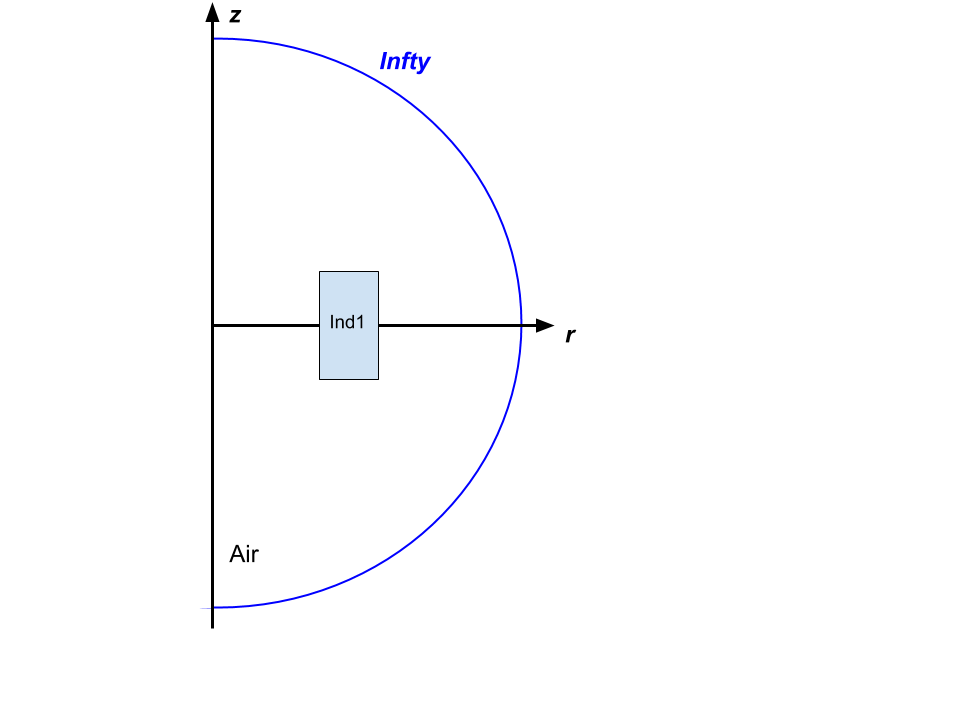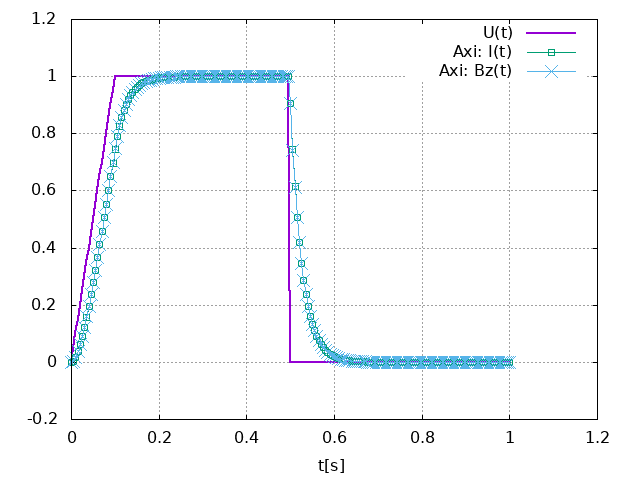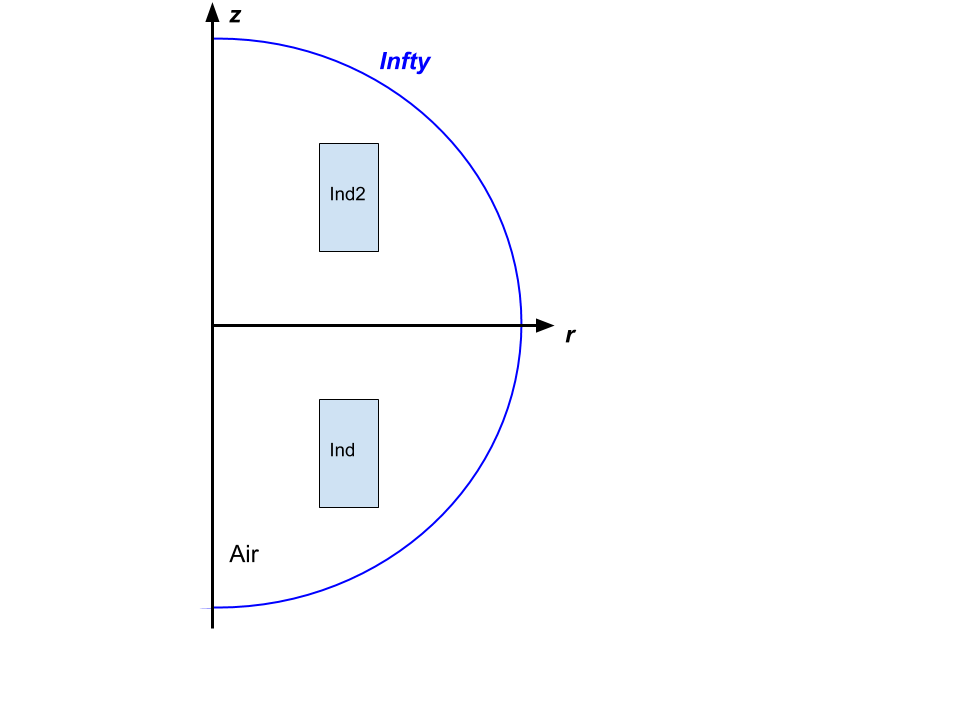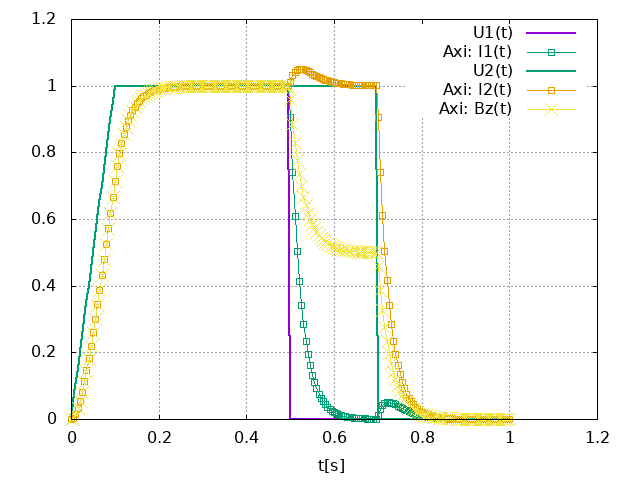Validations

1. Introduction

Two simple test cases are used to validate the formulation:

• a single magnet,

• a set of 2 magnets.

We will consider simple geometries for the magnets. They are modeled as rectangular cross section torus. This allows us to derive analytical expressions for the resistance $R$ and inductances $L$ of the magnets. For each case, we will model the powering and a powerfailure. The computed total current flowing into the magnets will be compared to the results obtained by a simple equivalent $RL$ circuit. The circuit may be either modeled by a simple ODE solver or using analytical methods (aka exponential Matrices). For other quantities, we will compared our simulation results with other Finite Element Solver. We have chosen getdp with a MQS formulation for axisymetric geometries.

NB: getdp model and test geometry are accessible here. To reproduce the results follow the procedure in this appendix.

2. A solenoidal magnet

2.1. Setup

The magnet is centered on the origin of the cylindrical frame as shown bellow.The geometry is defined by the following parameters:

Name Description Value Unit

$r_i$

75.

$mm$

$r_e$

100.2

$mm$

$h$

heigth

50.

$mm$

$r_\text{infty}$

500.

$mm$

The magnet is supposed to be made of some conducting amagnetic material:

Name Description Marker Value Unit

$\sigma$

electric conductivity

Ind

$58.e3$

$S.mm^{-1}$

$\mu_r$

relative magnetic permeability

Ind

$1$

The applied source electrical potential has the following form:

$V_D$ electrical potential $1*t/(0.1*\tau)$ $V$ $0 <= t < 0.1\,\tau$

$V_D$

electrical potential

1

$V$

$0.1 \, \tau <= t <= 0.5\,\tau$

$V_D$

electrical potential

0

$V$

$t>\tau$

The boundary conditions for the electromagnetic problem are:

Marker Type Value

$Oz$ Axis

Dirichlet

$\mathbf{0}$

Infty

Dirichlet

$\mathbf{0}$

2.2. Resistance $R$ and Self Inductance $L$

The resistance is defined as the ration of the applied electrical potential difference over the total current. In case of a rectangular cross section $(r_1,r_2) \times (z_1,z_2)$ torus, we can show that:

$R = \frac{2 \pi r_1 \rho}{r_1 ln(r_2/r_1)\,(z_2-z_1)}$

with $\rho=1/\sigma$ the resistivity of the material composing the torus. For details on this expression, see feelpp electric toolbox test case.

As for the self-inductance, we recall the defintion of the stored magnetic energy:

$E = \frac{1}{2} L I^2 = \frac{1}{2} \int_\mathbf{R^3} \mathbf{B} \times \mathbf{H}\, d\Omega$

to continue…​

2.3. Equivalent circuit model

From a macroscopic point of view, the studied system is simply equivalent to a $RL$ circuit modeled by:

$U(t) = R I(t) + L \, \frac{dI}{dt}$
Value Unit

$R$

$7.5313 10^{-6}$

Ohm

$L$

$1.9204 10^{-7}$

Henry

2.4. Results

The normalized computed electric potential, current and magnetic field at the Origin are plotted bellow:We use the expected values of the applied electric potential, current and magnetic field for the transient regime (aka t):

Value Unit

$V$

1

V

$I$

135069

A

$B_z(\mathbf{O})$

0.944

T

3. 2 solenoidal magnets

For sake of simplicity, we consider 2 solenoid magnets similar to the one described in previous section stacked as shown bellow.

insert a figure3.1. Mutual Inductance $M$

Obviously, the 2 magnets have the same resistance and self-inductance as they have the same geometry and are made of the same material. We only need then to compute the so-called mutual inductance $M$.

As before, we start wih the stored magnetic energy $E$ of the system:

$E = \frac{1}{2} \sum_k L_k I_k^2 + \sum_k \sum_{l \neq k} M_{k,l} I_k I_l$

to continue…​

3.2. Equivalent circuit model

The equivalent circuit is this time similar to a transformer circuit. Thus, it may be modeled as:

\begin{align} U_1(t) &=& R_1 I_1(t) + L_1 \, \frac{dI_1}{dt}+ M\,\frac{dI_2}{dt}, \\ U_2(t) &=& R_2 I_2(t) + M\, \frac{dI_1}{dt}+ L_2\,\frac{dI_2}{dt} \end{align}

In our case, we have $R_1=R_2$ and $L_1=L_2$.

Value Unit

$R$

$7.5313 10^{-6}$

Ohm

$L$

$1.9204 10^{-7}$

Henry

$M$

$2.6487 10^{-8}$

Henry

3.3. Results

The normalized computed electric potential, current and magnetic field at the Origin are plotted bellow:We use the expected values of the applied electric potential, current and magnetic field for the transient regime (aka t):

Value Unit

$V$

1

V

$I$

135069

A

$B_z(\mathbf{O})$

1.09325

T